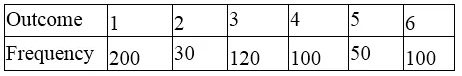## RD Sharma Class 9 Solutions Chapter 25 Probability MCQS

These Solutions are part of RD Sharma Class 9 Solutions. Here we have given RD Sharma Class 9 Solutions Chapter 25 Probability MCQS

Other Exercises

Mark the correct alternative in each of the following:

Question 1.
The probability of an impossible event is
(a) 1
(b) 0
(c) less than 0
(d) greater than 1
Solution:
The probability of an impossible event is 0 (b)

Question 2.
The probability on a certain event is
(a) 0
(b) 1
(c) greater than 1
(d) less than 1
Solution:
The probability of a certain event is 1 (b)

Question 3.
The probability of an event of a trial is
(a) 1
(b) 0
(c) less than 1
(d) more than 1
Solution:
The probability of an even of a trial is less than 1 (c)

Question 4.
Which of the following cannot be the probability of an event?
(a) $$\frac { 1 }{ 3 }$$
(b) $$\frac { 3 }{ 5 }$$
(c) $$\frac { 5 }{ 3 }$$
(d) 1
Solution:
The probability of an event is less than 1
$$\frac { 5 }{ 3 }$$ i.e .$$1\frac { 2 }{ 3 }$$ is not the probability

Question 5.
Two coins are tossed simultaneously. The probability of getting atmost one head is
(a) $$\frac { 1 }{ 4 }$$
(b) $$\frac { 3 }{ 4 }$$
(c) $$\frac { 1 }{ 2 }$$
(d) $$\frac { 1 }{ 4 }$$
Solution:
Total number of possible events (n) = 2 + 2 = 4
Number of events coming at the most 1 head (m) 2 times + 1 times = 3
Probability P(A) = $$\frac { m }{ n }$$ = $$\frac { 3 }{ 4 }$$ (b)

Question 6.
A coin is tossed 1000 times, if the probability of getting a tail is 3/8, how many times head is obtained?
(a) 525
(b) 375
(c) 625
(d) 725
Solution:
No. of times a coin is tossed (n) = 1000
Probability of getting a tail = $$\frac { 3 }{ 8 }$$
Let No. of tail come = x
Probability P(A) = $$\frac { m }{ n } =\frac { x }{ 1000 }$$
$$\frac { x }{ 1000 }$$ = $$\frac { 3 }{ 8 }$$
=> $$\frac { x }{ 1000 } =\frac { 3 }{ 8 }$$ => $$\frac { 3X1000 }{ 8 } =3X125$$
=> x = 375
∴ No. of heads are obtained = 1000 – 375 = 625 (c)

Question 7.
A dice is rolled 600 times and the occurrence of the outcomes 1, 2, 3, 4, 5 and 6 are given below:The probability of getting a prime number is
(a)$$\frac { 1 }{ 3 }$$
(b)$$\frac { 2 }{ 3 }$$
(c)$$\frac { 49 }{ 60 }$$
(d)$$\frac { 39 }{ 125 }$$
Solution:
Total number of times a dice is rolled (n) = 600Now total number of times getting a prime number i.e. 2, 3, 5, (m) = 30 + 120 + 50 = 200
Probability P(A) = $$\frac { m }{ n }$$ = $$\frac { 200 }{ 600 }$$ = $$\frac { 1 }{ 3 }$$ (a)

Question 8.
The percentage of attendance of different classes in a year in a school is given below:What is the probability that the class attendance is more than 75%?
(a) $$\frac { 1 }{ 6 }$$
(b) $$\frac { 1 }{ 3 }$$
(c) $$\frac { 5 }{ 6 }$$
(d) $$\frac { 1 }{ 2 }$$
Solution:
Percentage of attendance of different classesTotal attendance more than 75% (m) VIII,VII and VI = 3 classes
and total number of classes (n) = 6
Probability P(A) = $$\frac { 3 }{ 6 }$$ = $$\frac { 1 }{ 2 }$$.

Question 9.
A bag contains 50 coins and each coin is marked from 51 to 100. One coin is picked at random.
The probability that the number on the coin is not a prime number, is
(a) $$\frac { 1 }{ 5 }$$
(b) $$\frac { 3 }{ 5 }$$
(c) $$\frac { 2 }{ 5 }$$
(d) $$\frac { 4 }{ 5 }$$
Solution:
Total number of coins (n) = 50
Prime numbers between 51 to 100 are 53, 59, 6, 67, 71, 73, 79, 83, 89, 97 = 10
Numbers which are not primes (m) = 50 – 10 = 40
Probability P(A) = $$\frac { m }{ n }$$ = $$\frac { 40 }{ 50 }$$ = $$\frac { 4 }{ 5 }$$(d)

Question 10.
In a football match, Ronaldo makes 4 pals from 10 penalty kids. The probability of converting a penalty kick into a goal by Ronaldo,is
(a) $$\frac { 1 }{ 4 }$$
(b) $$\frac { 1 }{ 6 }$$
(c) $$\frac { 1 }{ 3 }$$
(d) $$\frac { 2 }{ 5 }$$
Solution:
No. of penalty kicks (n) = 10
No. of goal scored (m) = 4
Probability of converting a penally Into goals P(A) = $$\frac { 4 }{ 10 }$$ = $$\frac { 2 }{ 5 }$$(d)

Hope given RD Sharma Class 9 Solutions Chapter 25 Probability MCQS are helpful to complete your math homework.

If you have any doubts, please comment below. Learn Insta try to provide online math tutoring for you.